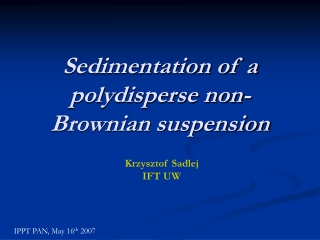DownloadDownload PresentationSedimentation of a polydisperse non-Brownian suspension

# Sedimentation of a polydisperse non-Brownian suspension

Download Presentation## Sedimentation of a polydisperse non-Brownian suspension

- - - - - - - - - - - - - - - - - - - - - - - - - - - E N D - - - - - - - - - - - - - - - - - - - - - - - - - - -
##### Presentation Transcript

1. Sedimentation of a polydisperse non-Brownian suspension Krzysztof Sadlej IFT UW IPPT PAN, May 16th 2007

2. Overview • Introduction and formulation of the problem • Discussion of Batchelors theory • Towards a correct and self-consistent solution • Results • Experimental data and discussion

3. Introduction • Slow sedimentation of hard spheres (radius app. 5-100 mm) in aviscous (η), non-compressible fluid. • No Brownian motion, Reynolds numbers small • Stokesian dynamics, stick boundaryconditions on the particles. g U2 U1 U4 U5 U3 Particle velocities are linearly proportional to the external forces acting on them: Configuration of all the particles The mobility matrix- a function of the particle positions. Scattering expansion in terms of one- and two-particle operators

4. Formulation of the problem Mean sedimentation velocity Sedimentation coefficient Stokes velocity Volume fraction of particles with radius aj and density rj

5. Discussion of Batchelors theory George Keith Batchelor (March 8, 1920 - March 30, 2000) • Monodisperse suspension (1972) • Random distribution of particles • S = -6.55 • Polydisperse suspension (1982) • Consideration of only two-particle dynamics

6. Batchelors results for non-Brownian suspensions • discontinuities in the form of the distribution function and the value of the sedimentation coefficient when calculating the limit of identical particles, • due to the existence of closed trajectories the solution of the problem does not exist for all particle sizes and densities. Monodisperse suspension: • S = -6.55 • Experimental results S= -3.9 (Ham&Homsy 1988)

7. Towards a correct and self-consistent solution Liouville Equation: Reduced distribution functions Cluster expansion of the mobility matrix BBGKY hierarchy

8. Correlation functions Hierarchy equations for h(s) Cluster expansion of mobility matrix Hierarchy contains infinite-range terms and divergent integrals!!

9. Solution • Low concentration limit – truncation of the hierarchy • Correlations in steady state must be integrable (group property ) • Finite velocity fluctuations (Koch&Shaqfeh 1992)

10. Describes correlation at the particle size length-scale. Equation derived based on the analysis of multi-particle hydrodynamic interactions and the assumption of integrability of correlations. Formula for this function and its asymptotic form may be found analytically. Explicit values for arbitrary particle separations and particle sizes/densities are calculated using multipole expansion numerical codes with lubrication corrections. The long-range structure scales with the particle volume fraction (b>0) Satisfies the Koch-Shaqfeh criterion for finite particle velocity fluctuations Once isotropy is assumed, the long-range structure function does not contribute to the value of the sedimentation coefficient. Screening on two different length scales

11. Explicit solution Functions describing two-particle hydrodynamic interactions Assymptotic form for large s:

12. Results Excess amount of close pairs of particles Function does not depend on the densities of particles Isotropic Well defined for all particle sizes and densities. The limit of identical particles is continuous.

13. Comparison do experiment Monodisperse suspension: S = -3.87 Batchelor: S = -6.55 Experimental results S= -3.9 (Ham&Homsy 1988) Polydisperse suspension Suspension of partcles with different radii and densities (D.Bruneau et al. 1990) Batchelors theory not valid.

14. Discussion Local formulation of the problem – well defined in the thermodynamic limit Multi-particle dynamics Self-consistent Comparison to experimental data very promising. Practical